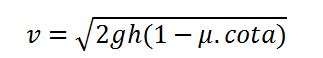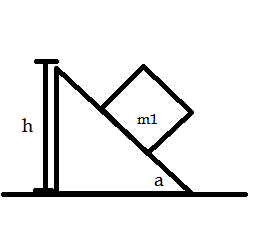# Final velocity of a rough block moving down a fixed wedge Calculator

This CalcTown calculator calculates the final velocity of a rough block moving down a fixed rough wedge from a height h.

*Please note that this formula is applicable only when a>tan-1(µ), otherwise the block will be at rest.

m
degree

#### Result

m/sClick here to view image

where

a=Angle of the wedge

h=Height of the wedge